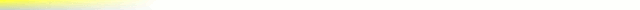# [mkgmap-dev] Bit operatons in mkgmap

From Steve Ratcliffe steve at parabola.me.uk on Wed Jul 20 22:30:24 BST 2016

```Hi Gerd

> I'd like to change this code in LinePreparer (and a copy in
> LinePreparerFilter)
>
>             // XXX: relies on the fact that java integers are 32 bit signed
>             final int offset = 8+shift;
> to
>
>             final int offset = (Integer.SIZE - 24) + shift;
>
> As you see I assume the value 8 is the result of 32 - 24.
> and I guess that 24 is the maximum resolution.
> Maybe we have a proper constant for that ?

Yes I think that it is supposed to be the difference between the 24
bit size of the Garmin word and the 32 bit java int.

But... does the following code that uses that value actually
work?

int dx = (lon - lastLong) << offset >> offset;
int dy = (lat - lastLat) << offset >> offset;

I assume that the intent is to sign extend the value, but the way the
value is calculated they should be already properly signed values.

If the dx, dy values are too big, they will be corrupted by the shift
operations. However the following assertions:

assert (dx == 0 && lon != lastLong) == false: ("delta lon too large: "
+  (lon - lastLong));
assert (dy == 0 && lat != lastLat) == false: ("delta lat too large: " +
(lat - lastLat));

claim to be a test for the delta being too large, but this cannot work
either.

But that is just looking at the code... I added a test to see if
there was ever a difference between the plain (lon - lastLong) value
and the double shifted value.  None found on compiling a UK map.

So I think that 'offset' can be removed entirely unless you can see a
case where it is needed?

..Steve

```